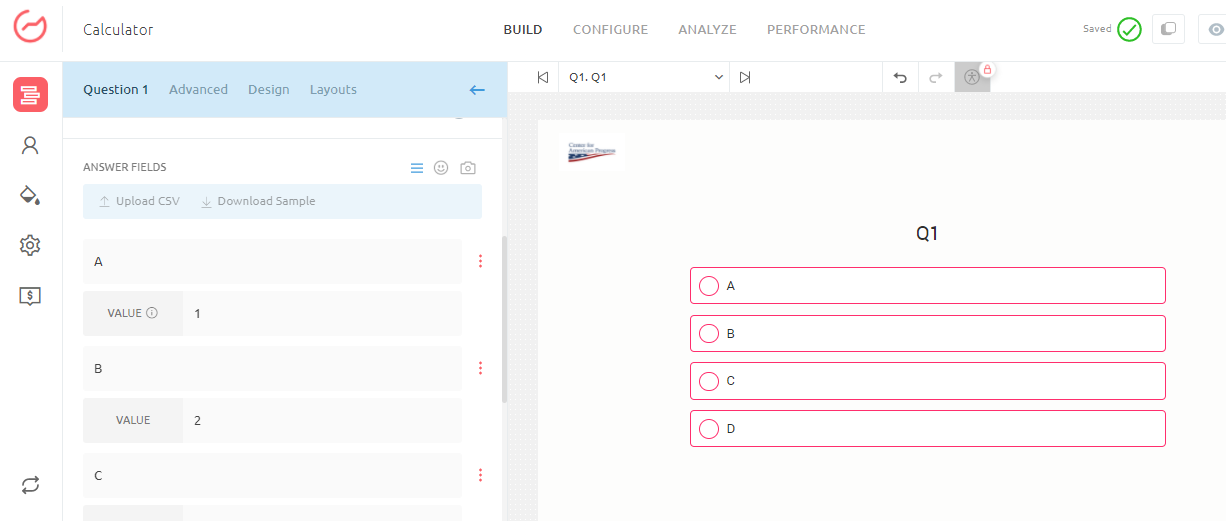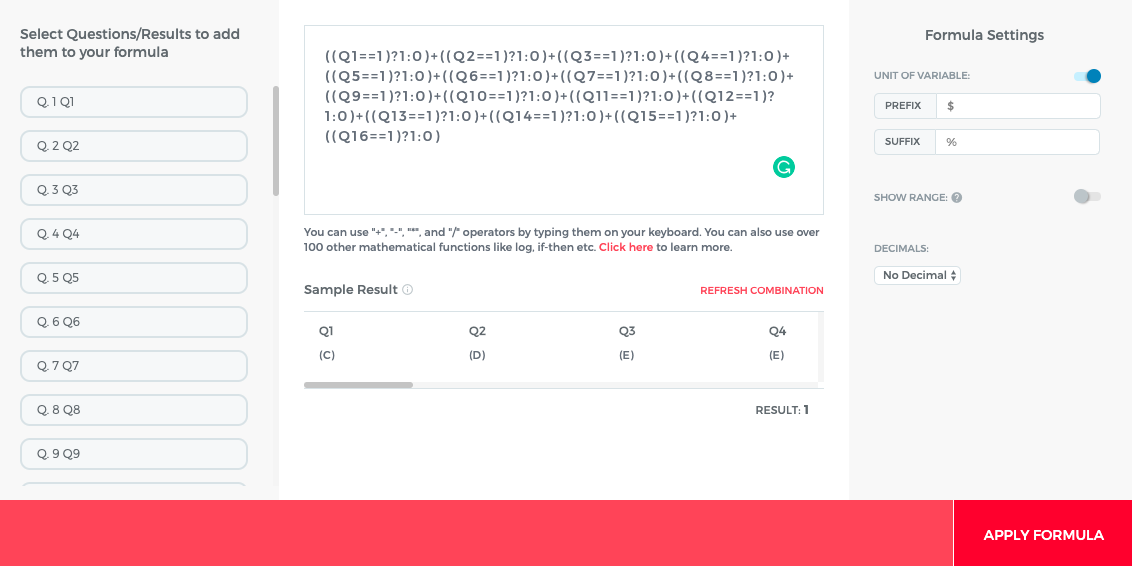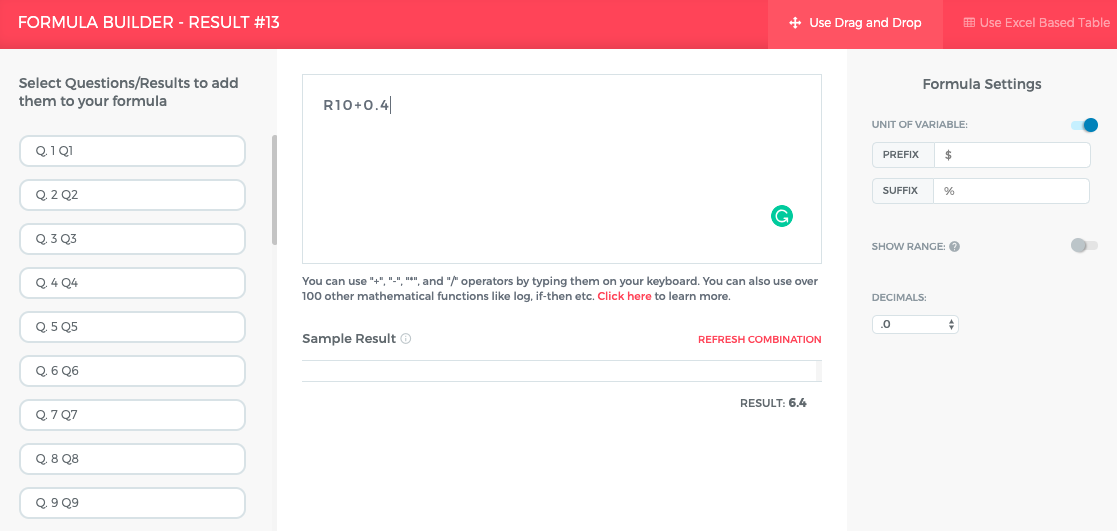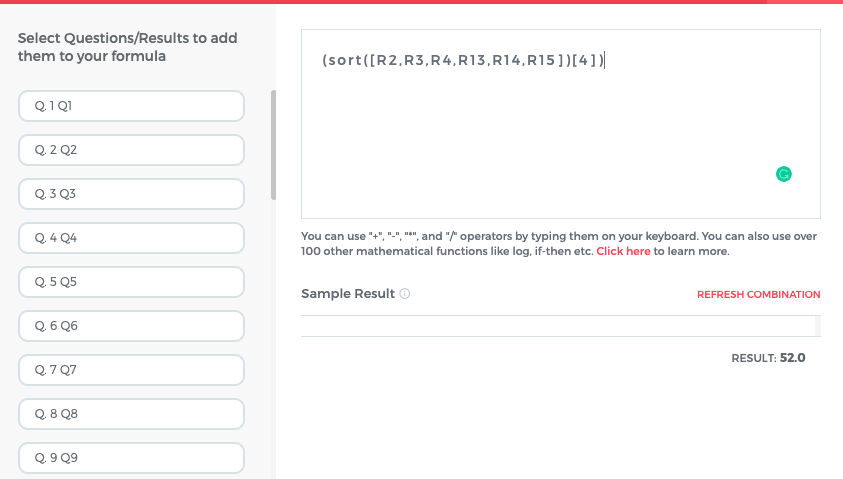# Implementing Sort Functionality in your Outgrow Calculator

In an Outgrow Calculator, you can now use the Sort functionality. This document will help you in learning more about the Sort function can be used in Outgrow.

# How to set up the Sort Function

1. Once you choose to create a Numerical Calculator from the main dashboard, you can add the questions and options in the calculator. You can assign 1,2,4,8 etc to each option every question.2. Once the questions and options are set up, the next and the important step that needs be taken is to create results for each outcome. While setting up the result you need to make sure that the formula for calculating result should be (((Q1==1)?1:0)+((Q2==1)?1:0) +... etc). This means that the result option is aligned to Outcome 1 and each question counts equally towards the final outcome. In case you want that each question should be counted as 2X, you need to change the value in the formula from 1 to 2. In this scenario the formula will become (((Q1==2)?1:0)+((Q2==2)?1:0)+... etc).3. In the 3rd step, you need to add .01, .02, .03, .04, etc to each result to handle tiebreakers.NOTE: In case the results are more than 10, then instead of adding .1, .2, etc, you need to add .01, .02, .03, etc.

4. Once the result and tiebreakers are set up, now you need to set the Sort Function to determine the position of each outcome. The formula for each outcome will be (sort([R2, R3, R4, R13, R14, R15])), etc. This will be the value at the top, so Conditional Messaging will have to be added to each value in the array. (sort([R2, R3, R4, R13, R14, R15])) will be the highest value in array and this will go on till the last value. You can follow it in descending order to get the other values.5. 5. In the end, you just need to sort R1 through R6 in highest to the lowest sequence. Once you figure out that which result will be in which position, you can set up Conditional Messaging accordingly.

Feel free to reach out to us at [email protected], or using the chat window at the bottom of the page, and we will be happy to assist you further.